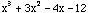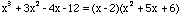Date: Sat, 17 Jan 1998 17:41:06 -0500
Subject: Synthetic Division

This question would be classified as secondary

Hi, I'm a ninth grade student doing a math project for school. I'm trying to learn about various ways of solving 3rd and 4th degree polynomial equations using the remainder theorem, the factor theorem, and synthetic division. The books I have all mention x - r, which I know nothing about, not even how to get it.

I would really appreciate any information on this. Thanks.

Dhruv

Hi Dhruv

Consider the polynomial p(x)=. If you substitute x=3 into this polynomial you get p(3)=27+27-12-12=30. Also if you divide (x-3) intoeither using synthetic division or long division you will get a remainder of 30. This is an illustration of the remainder theorem which is.

If p(x) is a polynomial, r is a number and p(r)=a then the remainder when dividing p(x) by (x-r) is also a.

In particular if p(r)=0 then the remainder is 0 and thus p(x)=(x-r)q(x) where q(x) is some other polynomial.

For the example above, p(x)=, you can see that p(2)=0 and thus=(x-2)q(x). You can find q(x) by dividing (x-2) into, either using long division or synthetic division. If you do so you find thatCheers,
Penny

Go to Math Central

To return to the previous page use your browser's back button.